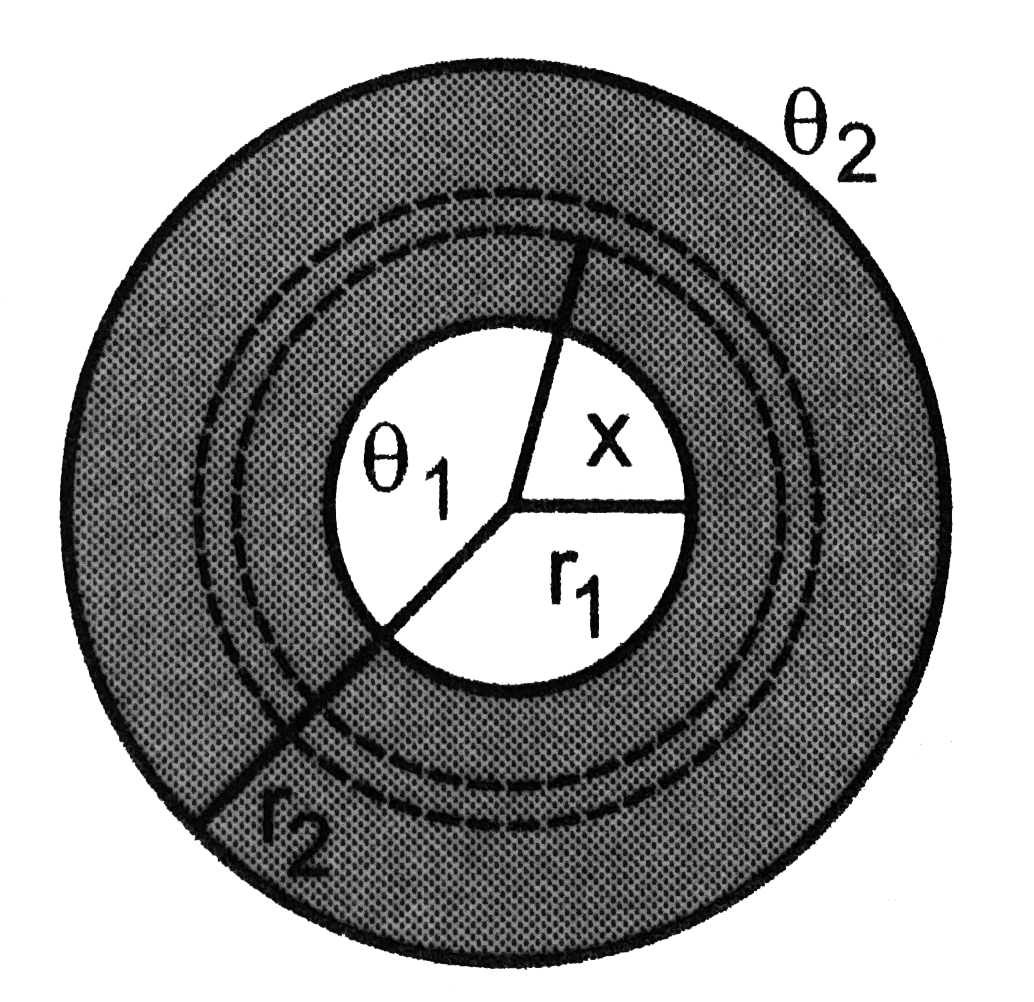# Two thin metallic spherical shells of radii r_(1) and r_(2) (r_(1)lt r_(2)) are placed with their centres coinciding. A material of thermal conduct

21 views
in Physics
closed
Two thin metallic spherical shells of radii r_(1) and r_(2) (r_(1)lt r_(2)) are placed with their centres coinciding. A material of thermal conductivity K is filled in the space between the shells. The inner shells. Is maintained at temperature theta_(1) .and the outer shell at temperature theta_(2) (theta_(1)lttheta_(2). Calculate the rate at which heat flows radially through the material.by (90.1k points)

Let us drow two spherical shells of radii x and x+dx concentric with the given system. Let the temperatures at these shells be theta and theta+dtheta respectively. The amount of heat flowing radially inward through the material between x and x+dx is
(DeltaQ)/(Deltat)=(K4pix^(2)d theta)/(dx) . thus, K4piint_(theta_(1))^(theta_(2))d theta=(DeltaQ)/(Deltat)int_(r1)^(R2)dx/x^(2) . or, K4pi(theta_(2)-theta_(1))=(DeltaQ)/(Deltat)((1)/R_(1)-(1)/R_(2)) . or, `(DeltaQ)/(Deltat)=(4piKr_(1)r_(2)(theta_(2)-theta_(1)))/(r_(2)-r_(1))